To compute F-values and degrees of freedom, let p be the rank of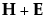. Let q be the rank of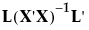, where the L matrix identifies elements ofassociated with the effect being tested. Let v be the error degrees of freedom and s be the minimum of p and q. Also let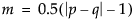and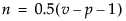.
Approximate F-statistics, gives the computation of each approximate F from the corresponding test statistic.
 Test Approximate F Numerator DF Denominator DF Wilks’ Lambda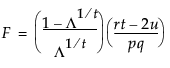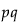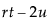Pillai’s Trace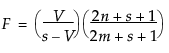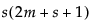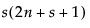Hotelling-Lawley Trace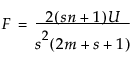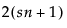Roy’s Max Root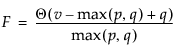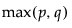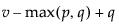Help created on 9/19/2017